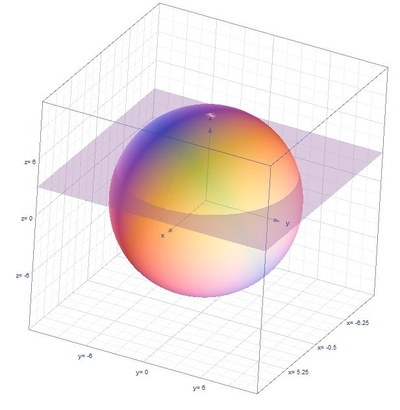# Find the volume of the solid bounded below by the plane z = 3 and above by the sphere...

## Question:

Find the volume of the solid bounded below by the plane {eq}z = 3{/eq} and above by the sphere {eq}x^2+y^2+z^2=9^2{/eq}. Write your answer as a reduced fraction.

## Volume of the Region:

For the volume of the solid we will be using the triple integrals formula in cylindrical coordinates which is {eq}V=\int_{\alpha }^{\beta }\int_{h_{1}(\theta )}^{h_{2}(\theta )}\int_{u_{1}(r\cos \theta ,r\sin \theta )}^{u_{2}(r\cos \theta ,r\sin \theta )}r\:f\left ( r\cos \theta ,r\sin \theta ,z \right )dzdrd\theta {/eq} and the conversion formulas are the following {eq}r^{2}=x^{2}+y^{2},\:x=r\cos \theta ,\:y=r\sin \theta {/eq}.

## Answer and Explanation:

Below is the graph,First let us find the limits, when {eq}z=3 {/eq}

{eq}x^{2}+y^{2}+\left ( 3 \right )^{2}=9^{2} {/eq}

{eq}x^{2}+y^{2}=72 {/eq}

Thus, the limits are the following

{eq}0\leq \theta \leq 2\pi ,\:0\leq r\leq 6\sqrt{2},\:3\leq z\leq \sqrt{81-r^{2}} {/eq}

The volume of the region is,

{eq}V=\int_{0}^{2\pi }\int_{0}^{6\sqrt{2}}\int_{3}^{\sqrt{81-r^{2}}}rdzdrd\theta {/eq}

Integrate with respect to {eq}z {/eq}

{eq}V=\int_{0}^{2\pi }\int_{0}^{6\sqrt{2}}\left [ z \right ]^{\sqrt{81-r^{2}}}_{3}rdrd\theta {/eq}

{eq}V=\int_{0}^{2\pi }\int_{0}^{6\sqrt{2}}\left ( \sqrt{81-r^{2}}-3 \right )rdrd\theta {/eq}

Integrate with respect to {eq}r {/eq}

{eq}V=\int_{0}^{2\pi }\left [ -\frac{1}{3}\left(81-r^2\right)^{\frac{3}{2}}-\frac{3r^2}{2} \right ]^{6\sqrt{2}}_{0}d\theta {/eq}

{eq}V=\int_{0}^{2\pi }126d\theta {/eq}

Integrate with respect to {eq}\theta {/eq}

{eq}V=126\left [ \theta \right ]^{2\pi }_{0} {/eq}

{eq}V=252\pi {/eq}

#### Learn more about this topic:Volumes of Shapes: Definition & Examples

from GMAT Prep: Tutoring Solution

Chapter 11 / Lesson 9
45K